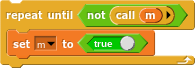# Recursion in c#?

does

while (m)
{
bool m = true;
}

work in c#?

that's not recursion

then what is it? im confused

i am confused too by how to answer your question, but that's not recursion, that's a while loop. recursion requires a function, and is when a function calls itself. here is an example of recursion:

int fibonacci(int n) {
if (n == 0 || n == 1) {
return n;
}
return fibonacci(n - 1) + fibonacci(n - 2)
}


i was trying to say thisbut in c#
while (m)
{
bool m = true;
}

Wouldn't that then be something like

bool m() {
//...
}

while (m()) {
m = true;
}


and that would result in an error. And calling a boolean would not work in Snap! either.

oh ok

This is the repeat while block.